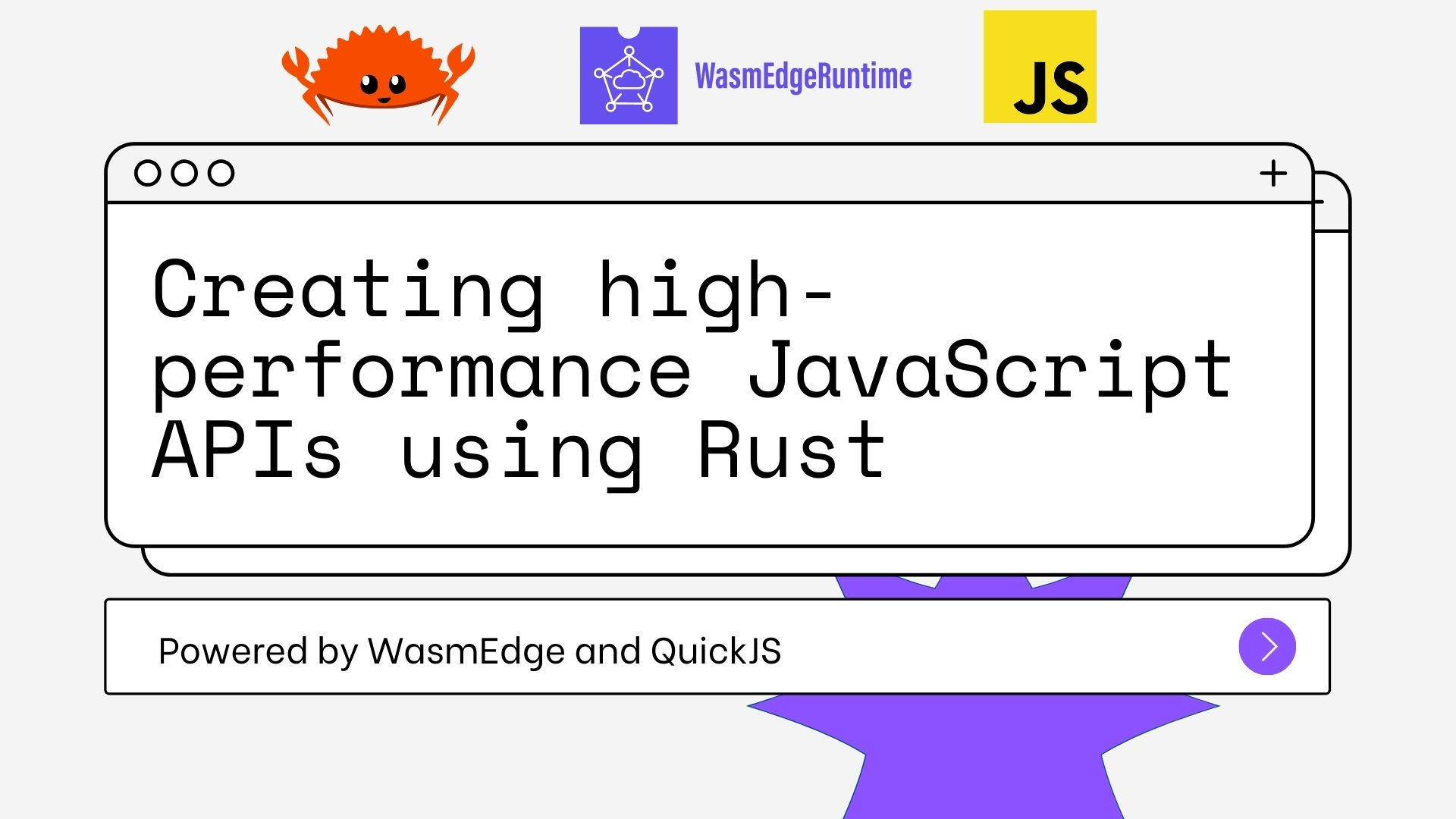WasmEdge 正在参加今年的 Hacktoberfest，为开源项目贡献4个 PR，就能获得 Hacktoberfest 提供的一件 T shirt 。

WasmEdge 将 Rust 的性能和 JavaScript 的易用性结合在一起

``````\$ git clone https://github.com/second-state/wasmedge-quickjs
\$ cd examples/embed_js
``````

`embed_js` demo 展示了几个关于如何在 Rust 中嵌入 JavaScript 的不同示例。 你可以按如下方式构建和运行所有示例。

``````\$ cargo build --target wasm32-wasi --release
\$ wasmedge --dir .:. target/wasm32-wasi/release/embed_js.wasm
``````## 创建一个 JavaScript 函数 API

``````fn run_rust_function(ctx: &mut Context) {

struct HelloFn;
impl JsFn for HelloFn {
fn call(_ctx: &mut Context, _this_val: JsValue, argv: &[JsValue]) -> JsValue {
println!("hello from rust");
println!("argv={:?}", argv);
JsValue::UnDefined
}
}

...
}
``````

``````fn run_rust_function(ctx: &mut Context) {
...

let f = ctx.new_function::("hello");
ctx.get_global().set("hi", f.into());
let code = r#"hi(1,2,3)"#;
let r = ctx.eval_global_str(code);
println!("return value:{:?}", r);
}

``````

``````hello from rust
argv=[Int(1), Int(2), Int(3)]
return value:UnDefined
``````

## 创建 JavaScript 对象 API

``````fn rust_new_object_and_js_call(ctx: &mut Context) {

struct ObjectFn;
impl JsFn for ObjectFn {
fn call(_ctx: &mut Context, this_val: JsValue, argv: &[JsValue]) -> JsValue {
println!("hello from rust");
println!("argv={:?}", argv);
if let JsValue::Object(obj) = this_val {
let obj_map = obj.to_map();
println!("this={:#?}", obj_map);
}
JsValue::UnDefined
}
}

``````

``````    let mut obj = ctx.new_object();
obj.set("a", 1.into());
obj.set("b", ctx.new_string("abc").into());

let f = ctx.new_function::("anything");
obj.set("f", f.into());

``````

``````ctx.get_global().set("test_obj", obj.into());
``````

``````let code = r#"
print('test_obj keys=',Object.keys(test_obj))
print('test_obj.a=',test_obj.a)
print('test_obj.b=',test_obj.b)
test_obj.f(1,2,3,"hi")
"#;

ctx.eval_global_str(code);
}
``````

``````test_obj keys= a,b,f
test_obj.a= 1
test_obj.b= abc
hello from rust
argv=[Int(1), Int(2), Int(3), String(JsString(hi))]
this=Ok(
{
"a": Int(
1,
),
"b": String(
JsString(
abc,
),
),
"f": Function(
JsFunction(
function anything() {
[native code]
},
),
),
},
)
``````

## 一个完整的 JavaScript 对象 API

``````\$ cargo build --target wasm32-wasi --release
\$ wasmedge --dir .:. target/wasm32-wasi/release/embed_rust_module.wasm

``````

``````mod point {
use wasmedge_quickjs::*;

#[derive(Debug)]
struct Point(i32, i32);

struct PointDef;

impl JsClassDef for PointDef {
const CLASS_NAME: &'static str = "Point\0";
const CONSTRUCTOR_ARGC: u8 = 2;

fn constructor(_: &mut Context, argv: &[JsValue]) -> Option {
println!("rust-> new Point {:?}", argv);
let x = argv.get(0);
let y = argv.get(1);
if let ((Some(JsValue::Int(ref x)), Some(JsValue::Int(ref y)))) = (x, y) {
Some(Point(*x, *y))
} else {
None
}
}

fn proto_init(p: &mut JsClassProto) {
struct X;
impl JsClassGetterSetter for X {
const NAME: &'static str = "x\0";

fn getter(_: &mut Context, this_val: &mut Point) -> JsValue {
println!("rust-> get x");
this_val.0.into()
}

fn setter(_: &mut Context, this_val: &mut Point, val: JsValue) {
println!("rust-> set x:{:?}", val);
if let JsValue::Int(x) = val {
this_val.0 = x
}
}
}

struct Y;
impl JsClassGetterSetter for Y {
const NAME: &'static str = "y\0";

fn getter(_: &mut Context, this_val: &mut Point) -> JsValue {
println!("rust-> get y");
this_val.1.into()
}

fn setter(_: &mut Context, this_val: &mut Point, val: JsValue) {
println!("rust-> set y:{:?}", val);
if let JsValue::Int(y) = val {
this_val.1 = y
}
}
}

struct FnPrint;
impl JsMethod for FnPrint {
const NAME: &'static str = "pprint\0";
const LEN: u8 = 0;

fn call(_: &mut Context, this_val: &mut Point, _argv: &[JsValue]) -> JsValue {
println!("rust-> pprint: {:?}", this_val);
JsValue::Int(1)
}
}

}
}

struct PointModule;
impl ModuleInit for PointModule {
fn init_module(ctx: &mut Context, m: &mut JsModuleDef) {
}
}

pub fn init_point_module(ctx: &mut Context) {
ctx.register_class(PointDef);
ctx.register_module("point\0", PointModule, &["Point\0"]);
}
}
``````

``````use wasmedge_quickjs::*;
fn main() {
let mut ctx = Context::new();
point::init_point_module(&mut ctx);

let code = r#"
import('point').then((point)=>{
let p0 = new point.Point(1,2)
print("js->",p0.x,p0.y)
p0.pprint()
try{
let p = new point.Point()
print("js-> p:",p)
print("js->",p.x,p.y)
p.x=2
p.pprint()
} catch(e) {
print("An error has been caught");
print(e)
}
})
"#;

ctx.eval_global_str(code);
ctx.promise_loop_poll();
}
``````

``````rust-> new Point [Int(1), Int(2)]
rust-> get x
rust-> get y
js-> 1 2
rust-> pprint: Point(1, 2)
rust-> new Point []
js-> p: undefined
An error has been caught
TypeError: cannot read property 'x' of undefined
``````